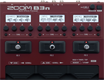# Factory : SynBass

Discussion in 'Zoom B3n' started by Tonelib, May 20, 2018.

1. Factory : SynBassDevice: Zoom B3n
Firmware: 2.00

Name on device: SynBass
Optimized for: Phones/Speaker

Effects chain:This bright synth bass sound uses Z-Syn.

Effect: "ZNR" (Dynamics), active - "yes"
"Detect" = GTRIN
"Depth" = 100
"Threshold" = 46
"Decay" = 0

Effect: "Z-Syn" (Sfx), active - "yes"
"Frequency" = 4
"Range" = 14
"Decay" = 85
"Resonance" = 13
"Wave" = SQR
"Tone" = 0
"Balance" = 44
"Volume" = 100
"Frequency" = 4
"Range" = 9
"Decay" = 72
"Resonance" = 18
"Wave" = SQR
"Tone" = 5
"Balance" = 46
"Volume" = 100
"Parameter set" = A

Effect: "Ba Octave" (Modulation), active - "yes"
"Oct" = 16
"Lo" = 2
"Hi" = 8
"Dry" = 100

Effect: "PitchSHFT" (Modulation), active - "yes"
"Shift" = 7
"Fine" = 0
"Tone" = 7
"Balance" = 10

Effect: "Splitter" (Filter), active - "yes"
"Frequency" = 125Hz
"Lo" = 30
"Hi" = 44
"Volume" = 45

Patch Volume: 110